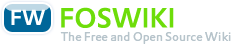# Course Processing of measured signals

Responsible: Prof. Dr. Stoll

## Course

### Meets requirements of following modules(MID)

• in active programs

### Course Organization

Version
created 2013-05-28
VID 2
valid from WS 2012/13
valid to
Course identifiers
Long name Processing of measured signals
CID F07_MSV
CEID (exam identifier) 766

Contact hours per week (SWS)
Lecture 2
Exercise (unsplit) 2
Exercise (split)
Lab 1
Project
Seminar
Tutorial(voluntary)
Total contact hours
Lecture 30
Exercise (unsplit) 30
Exercise (split)
Lab 15
Project
Seminar
Tutorial (voluntary)
Max. capacity
Exercise (unsplit)
Exercise (split) 40
Lab 16
Project
Seminar

Total effort (hours): 150

• German

#### Prerequisites

• Electronics 1, 2
• Measurement technique

• Hoffmann J., Taschenbuch der Messtechnik, Hanser
• Lindner et. al., Taschenb. der Elektrotechnik und Elektronik, fv Leipzig-Köln

#### Instructors

• Prof. Dr. Stoll

• none

#### Transcipt Entry

Processing of measured signals

### Assessment

Type
wE written Exam

Total effort [hours]
wE written Exam

Frequency: 2/Jahr

## Course components

### Lecture/Exercise

#### Objectives

##### Lerninhalte (Kenntnisse)
• Industrial measurement chain
• Properties of a measuring device
• Ideal properties
• Real properties
• Precision
• Zero point and span error
• Error of linearity
• Linearization
• Error by hysteresis
• Influence quantities
• Dynamic properties
• Analog processing of measured signals
• Ideal operational amplifier
• Properties and basic circuits
• Design and calculation of OP-circuits
• Interfaces between measurement devices
• Potential levels
• Supply
• 4...20mA - interface
• Galvanic isolation
• Controlled current sources
• Current controlled current sources
• Voltage controlled current sources
• Differential amplifier
• Common mode rejection
• Input impedance
• Instrumentation amplifier
• Real operational amplifier
• Small signal macromodel
• Effect of influence quantities
• Effect of drift
• Nonlinear devices
• Multiplier
• Carrier frequency technique
• Electronic analog switches
• Comparators
• Voltage controlled oscillators (VCO)
• Phase-Locked-Loop (PLL)
• Analog-Digital-Converter
• Digital processing of measured signals
• Test signals
• Periodic signals
• Description in time domain
• Description by a period
• Analytic signal
• Description with harmonic functions
• Description in frequency domain
• Harmonic analysis as approximation problem
• Description by sinus- and cosinus series
• Amplitude- and phase spectrum
• Compex Fourier series
• Frequency response, linear distortion
• Root mean square value (RMS), Parseval relation
• Transients
• Description in time domain
• Description in frequency domain
• Fourier transform
• Frequency response
• spectral energy density, Parseval relation
• Auto- and crosscorrelation function
• crosscorrelation function
• autocorrelation function
• Wiener-Khinchin relations
• Transients with infinite energy
• Stationary random signals
• Statistic distribution function
• RMS value, spectral power density, Parseval relation
• Correlation function and Wiener-Khinchin relation
• Crosscorrelation und crossspectrum
• Autocorrelation and spectral power density
• Coherence function
• Frequency response
• Convolution
• Discretisation
• Discretisation in time domain
• Sampling in time domain
• Reconstruction of analog function of time from samples
• Discretisation in frequency domain
• Sampling in frequency domain
• Reconstruction of analog Fourier transform from samples
• Discrete Fourier transform
• Time windows
• Relations between 2 discrete signals
• Discrete crosscorrelation function
• Discrete convolution
• Measurements with stationary, ergodic random signals
• Power density spectrum
• Coherence function
• Frequency response
• Z-Transformation
• Short introduction
• Z-Transform of a delay element
• Discrete convolution
• Digital filters
• FIR-Filter
• IIR-Filter (Recursive digital systems)
• Design of digital filters
• Stability of linear, timediscrete systems
• Digital-Analog-converter
• in measuring stations
• LabView
• System analysis
• System analysis in frequency domain
• Mathematical models
• Physical models
• Measuring of physical parameters
• System analysis in time domain
• Mathematical models
• Physical models
• Measuring of physical parameters
• in transmitters
• Smart transmitter

##### Acquired Skills
• Specifying differences between measuring chains in
• Laboratory / Science
• Embedded systems
• Industry
• Students are able to choose, design and optimize appropriate electronic devices to measure a certain quantity in an industrial environment
• Students are able to apply analog and digital processing of measured signals and they can evaluate their suitability and behaviour
• Students are able to decide, wether to solve a problem in processing measured signals with analog or digital methods
• Students can choose electronic measurment processes and circuits for transmitters
• Students can capture measurement data computer-based and process them with methods, which are appropriate for PC, microcontroller, digital signal processors and embedded systems

##### Operational Competences
• Solving exercises

Type
wE written Exam

Frequency: 2/Jahr

### Lab

#### Objectives

##### Lerninhalte (Kenntnisse)
• Experiment 1: Transmitters use and application, wiring, calibration, electric interfaces, Hart protocol, standardized user interface, Field Device Tool (FDT) with Device Type Manager (DTM) e.g.. PACTware or VDI/VDE-Richtlinie 2187
• Experiment 2: Design and build-up of a 4...20mA-transmitter for temperature on a circuit board, test, calibration, measurement of characteristic and influence of ambient temperature
• Experiment 3: RMS value. Comparison of different kinds of signal processing: electric (moving iron instrument), analog physical (thermo converter), analog electronic (multimeter), digital (DSO) and manual calculation
• Experiment 4: Digital data acquisition and -processing (DAQ). Analog measurement signals are captured with a PC, processed and measured values are output. Software LabView (NI) with virtual instruments (VI). Measurement devices with different interfaces are used: on board standard PC, USB, RS232, GPIB, Ethernet
• Experiment 5: Analysis of dynamic systems, measurement of frequency response with stochastic signals, estimation of model parameters. Generation of test signals with an arbitrary waveform generator. DAQ with DSO and sound card. Controlled by LabView (NI)

##### Acquired Skills
• Recognition of necessary knowledge and acquirement
• Processing of measured signals with different methods
• electric
• analog physical
• analog electronic
• digital
• Planning of systematic implementation
• Adjusting experimental procedure to unexpected situations
• Interpretation of test results, evaluation
• Abstract of results
• Delivery of report on the due date

##### Operational Competences
• Experimental procedureDeutsch English